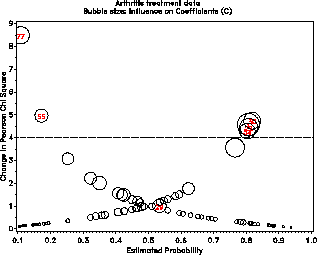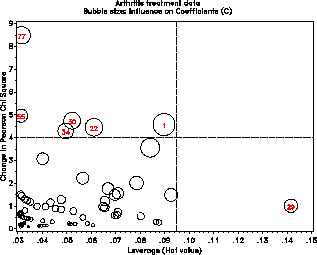inflogis Influence plots for logistic regression inflogis

# SAS Macro Programs: inflogis

\$Version: 1.3 (20 Mar 2003)
Michael Friendly
York University

## The inflogis macro (get inflogis.sas)

### Influence plots for logistic regression

The INFLOGIS macro produces influence plots for a logistic regression model. The plot shows a measure of badness of fit for a given case (DIFDEV or DIFCHISQ) vs. the fitted probability (PRED) or leverage (HAT), using an influence measure (C or CBAR) as the size of a bubble.

### Method

The macro fits the logistic model and obtains an output data set containing the diagnostic quantities which are plotted.

## Usage

inflogis is a macro program. Values must be supplied for the Y= and X= parameters.

The arguments may be listed within parentheses in any order, separated by commas. For example:

```   %inflogis(Y=responsevar, X=independentvars, ..., )
```

### Parameters

Y=
Name of criterion variable
X=
Names of predictors
ID=
Name of observation ID variable (char)
DATA=_LAST_
The name of the input dataset. If not specified, the most recently created dataset is used.
GY=DIFDEV
Ordinate for plot: Specify one or more of DIFDEV or DIFCHISQ
GX=PRED
Abscissa for plot: PRED or HAT
BUBBLE=C
Influence measure the bubble is made proportional to: specify either BUBBLE=C or CBAR
LABEL=INFL
Points to be labelled with the value of the ID variable in the plot: ALL, NONE, or INFL. The choice INFL causes only influential points to be labelled.
LSIZE=1.5
Observation label size. The height of other text (axis labels, etc.) is controlled by the HTEXT= goption
LCOLOR=BLACK
Observation label color
LPOS=5
Observation label position, using the position values understood by the Annotate facility.
BSIZE=10
bubble size scale factor
BSCALE=AREA
Sacle for the bubble size. AREA makes the bubble area proportional to the influence measure; RADIUS makes the bubble radius proportional to influence.
BCOLOR=BLACK
bubble color
REFCOL=BLACK
color of reference lines
REFLIN=33
line style for reference lines; 0->NONE
LOPTIONS=NOPRINT
NAME=INFLOGIS
Name for graphic catalog entry
GOUT=
Name of graphics catalog

### Example

The example below displays an influence plot for a logistic regression model predicting outcome of a treatment for arthritis pain, based on Sex, Treatment, and Age.

The plots display the Chi-square difference when the observation is deleted vs. both thepredicted probability of succesful outcome and the leverage with the bubble size proportional to the Cook's D measure of influence.

```%include macros(inflogis);        *-- or include in an autocall library;
%include data(arthrit);

%inflogis(data=arthrit,
y=better,
x=_sex_ _treat_ age,
id=case,
gy=DIFCHISQ,
gx=PRED HAT,
lcolor=RED, bsize=14
);
```